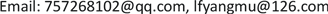K-Means聚类，股票分析，投资建议, K-Means Cluster Stock Analysis Investment Recommen-dations1. 前言

2. 研究目的与方法2.1. 研究目的

2.2. 研究方法

3. 经典K-means聚类算法

∪ j = 1 m C j = Ω (1)

C i ∩ C j = ∅ , i , j = 1 , 2 , ⋯ , m (3)

J = ∑ j = 1 m ∑ i = 1 n j d ( x i , z j ) , x i ∈ C j (4)

I：从数据集 Ω 中随机选择 m 个样本作为初始聚心；

II：根据类 C j ( j = 1 , 2 , ⋯ , m ) 中所含样本的均值，计算每个样本到各类聚心 z j 的距离，把其归到距离最小的类；

III：重新计算类 C j ( j = 1 , 2 , ⋯ , m ) 的聚心 z j ；

IV：计算本次迭代的聚类质量评估函数 J ( t ) 并和上次迭代的聚类质量评估函数 J ( t − 1 ) 比较；若两者之差满足阈值则算法结束，否则转到II继续执行。

4. 数据来源

5. 聚类结果分析

Classification results of K-means clusterin

6. 结束语